Next: Vertical Stability of Floating Up: Hydrostatics Previous: Buoyancy

# Equilibrium of Floating Bodies

Consider the situation described in the previous section. Suppose that the fluid contained withinis replaced by a partially submerged solid body whose outer surface corresponds to. Furthermore, suppose that this body is in mechanical equilibrium with the surrounding fluid (i.e., it is stationary, and floating on the surface of the water). It follows that the pressure distribution in the surrounding fluid is unchanged [because the force balance criterion (2.3) can be integrated to give the pressure distribution (2.4) at all contiguous points in the fluid, provided that the fluid remains in mechanical equilibrium]. We conclude that the net surface force acting acrossis also unchanged (because this is directly related to the pressure distribution in the fluid immediately surrounding), which implies that the buoyancy force acting on the floating body is the same as that acting on the displaced water: that is, the water that previously occupied. In other words, from Equation (2.12),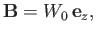(2.14)

where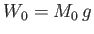and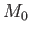are the weight and mass of the displaced water, respectively. The fact that the buoyancy force is unchanged also implies that the vertical line of action of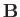passes through the center of gravity,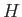(say), of the displaced water. Incidentally,is generally known as the center of buoyancy.

A floating body of weightis acted upon by two forces: namely, its own weight,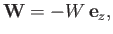(2.15)

and the buoyancy force,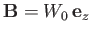, due to the pressure of the surrounding water. Of course, the line of action of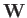passes through the body's center of gravity,(say). So as to remain in equilibrium, the body must be subject to zero net force and zero net torque. The requirement of zero net force yields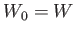. In other words, in equilibrium, the weight of the water displaced by a floating body is equal to the weight of the body, or, alternatively, in equilibrium, the magnitude of the buoyancy force acting on a floating body is equal to the weight of the displaced water. This famous result is known as Archimedes' principle, after Archimedes of Syracuse (c. 278-212 BCE). The requirement of zero net torque implies that, in equilibrium, the center of gravity,, and center of buoyancy,, of a floating body must lie on the same vertical straight-line.

Consider a floating body of mass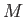and volume. Letbe the body's mean mass density. Archimedes' principle implies that, in equilibrium,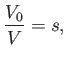(2.16)

where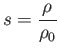(2.17)

is termed the body's specific gravity. (Recall, that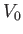is the submerged volume, and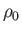the mass density of water.) We conclude, from Equation (2.16), that the volume fraction of a floating body that is submerged is equal to the body's specific gravity. Obviously, the specific gravity must be less than unity, because the submerged volume fraction cannot exceed unity. In fact, if the specific gravity exceeds unity then it is impossible for the buoyancy force to balance the body's weight, and the body consequently sinks.

Consider a body of volumeand specific gravitythat floats in equilibrium. It follows, from Equation (2.16), that the submerged volume is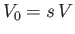. Hence, the volume above the waterline is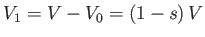. Suppose that the body is inverted such that its previously submerged part is raised above the waterline, and vice versa: that is,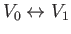. According to Equation (2.16), the body can only remain in equilibrium in this configuration if its specific gravity is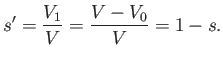(2.18)

We conclude that for every equilibrium configuration of a floating body of specific gravitythere exists an inverted equilibrium configuration for a body of the same shape having the complementary specific gravity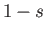.Next: Vertical Stability of Floating Up: Hydrostatics Previous: Buoyancy
Richard Fitzpatrick 2016-03-31